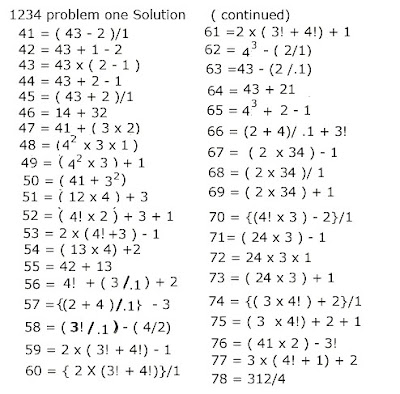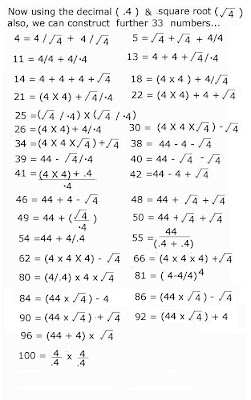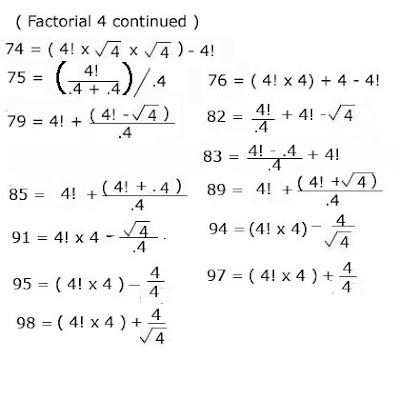# untamed

"Where the mind is without fear and the head is held high; Where knowledge is free; Where the world has not been broken up into fragments by narrow domestic walls; Where words come from the depth of truth; Where tireless striving stretches its arms toward perfection; Where the clear stream of reason has not lost its way into the dreary desert sand of dead habit; Into that heaven of freedom it should be our intent. ( my adaption from Rabindranath Tagores famous lines)

## Monday, September 21, 2009

### Arithmetic Recreations

Arithmetic Recreations
Before we tackle the FOUR FOURS Problem which we carry out in my next session it is easier to start with the 1234 problem which has the same rules. Here we have to express all the numbers from 1 to 100 using the digits only once in each expression. Remember all the 4 digits must be used only once to form the number. The notations allowable are Multiplication (x), division (/), addition (+) and subtraction (-). We also define that the only other symbols to attach to a digit are the decimal (.), factorial (! - For example 4! = 4 x 3 x 2 x 1 =24), and (exponential - a digit raised to the power of another digit). There are several solutions and to make it a little different I am excluding the square root function in my solution.## Wednesday, September 16, 2009

### Mathematical Recreations

Whilst reminiscing what I did 60 years back (I am 81 now), in my spare time when I was alone by myself and when there was no TV, mobile phone, computer, Internet or even a ball point pen I found that I amused myself with Mathematical recreations, reading such books and using a Venus 1B Pencil to make notes or solve a problem. Of course when I had friends or family we played Cricket Tennis etc and when indoors, Draughts, Monopoly, Cards, etc. Even today I am intrigued by the subject though I find it more difficult or impossible to solve many problems that I used to earlier. One such Arithmetical Recreation was the FOUR FOURS PROBLEM. I had solved the problem and had written in my book which I was intending publishing one day. Now when I googled “Four Fours” on my computer I was surprised to find many solutions (some wrong and inelegant). And even Wikipedia covered the problem. Nevertheless I wished to blog my solution.

THE FOUR FOURS PROBLEM

The objective of the four fours problem is to find a mathematical expression for every integer from 1 to 100; using only common mathematical symbols and exactly four fours (no other digits are allowed). The rider I add is to express these in the simplest possible manner.

Operations and symbols that are allowed include the four arithmetic operations (+, ×, -, /), concatenation (e.g. the use of 44), decimal points (e.g. 4.4), powers (e.g. 44), square roots, factorials (4! - which is 4 x 3 x2 x 1 =24), and overbars for repeating decimal (e.g. .4 with an over bar to express 0.44444….) – I found that to express 6 numbers I had to resort to this.)